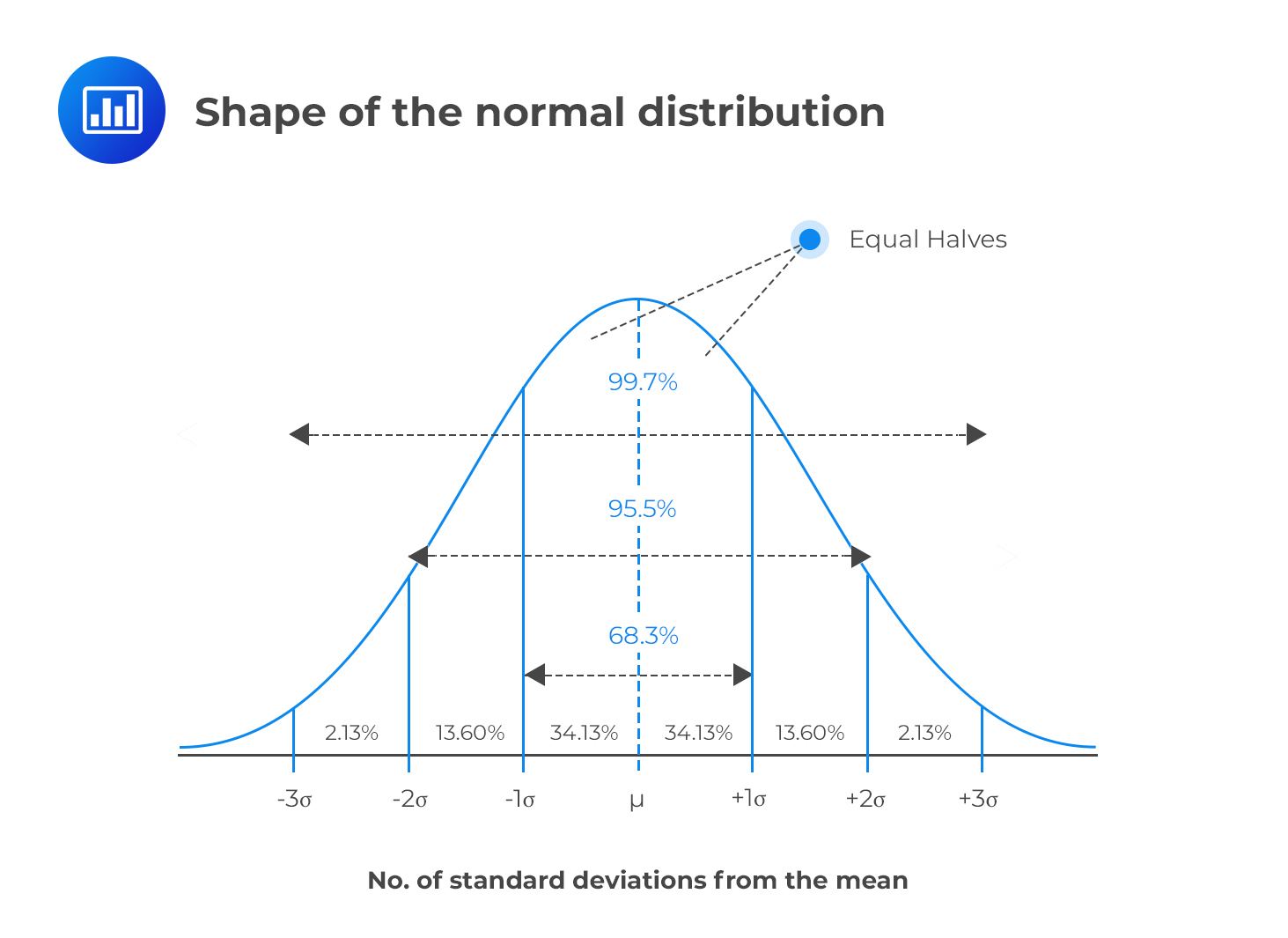Limited Time Offer: Save 10% on all 2021 and 2022 Premium Study Packages with promo code: BLOG10    Select your Premium Package »Normal Distribution and Confidence Intervals

A confidence interval (CI) gives an “interval estimate” of an unknown population parameter such as the mean. It gives us the probability that the parameter lies within the stated interval (range). The precision or accuracy of the estimate depends on the width of the interval.

For us to define a $$100(1 – α)%$$ confidence interval for $$θ$$, we must specify two random variables $$θ_1(X)$$ and $$θ_2(X)$$ such that $$P(θ_1(X) < θ < θ_2(X)) = 1 – α$$.

The most common value for $$α$$ is 0.05, which leads us to a 95% confidence interval. As such, $$P(θ_1(X) < θ < θ_2>(X)) = 0.95$$ specifies $$θ_1(X)$$ and $$θ_2(X)$$ such that there is a 95% chance of finding the true value of $$θ$$ in the interval. Alternatively, we could say that 5% of the realizations of such intervals would not contain the true value of $$θ$$.

The Importance of a Confidence Interval

It is not always possible to know the exact values of the population mean or the population standard deviation. This is because most populations are too large to allow data collection from every subject. In fact, collection of data from every subject in a large population is not only economically unviable but also very time-consuming. This makes confidence intervals very important.

Confidence intervals can also be used to predict the value of a given parameter. We usually assume that the underlying random variable has a normal distribution. In this regard, the central limit theorem (the assertion that most distributions tend to adopt a normal distribution when n is large) is a very important tool.

The following statements are true for any random variable that assumes a normal distribution:

1. 50% CI: approximately 50% of all observations fall in the range $$μ ± \left(\frac{2}{3}\right)σ$$.
2. 68% CI: approximately 68.3% of all the observations fall in the range $$μ ± σ$$.
3. 95% CI: approximately 95.5% of all observations fall in the interval $$μ ± 2σ$$.
4. 99% CI: approximately 99.7% of all the observations fall in the interval $$μ ± 3σ$$.Note to candidates: The words “interval” and “range” have been used interchangeably in this context.

Example: Confidence Interval

A financial analyst encounters a client whose portfolio return has a mean yearly return of 24% and a standard deviation of 5%. Assuming a normal distribution, the 50% confidence interval for the expected return is closest to:

Solution

\begin{align*} \text{Confidence interval at 50%} & = \left\{ 0.24 – \cfrac {2}{3} \times 0.05, 0.24 + \cfrac {2}{3} \times 0.05 \right\} \\ & = \left\{ 0.207, 0.273 \right\} \\ \end{align*}

Question

A financial analyst encounters a client whose portfolio return has a mean yearly return of 24% and a standard deviation of 5%. Assuming a normal distribution, a 99% confidence interval for the expected return is closest to:

1. {0.08, 0.49}.
2. {0.09, 0.39}.
3. {0.09, 0.9}.

Solution

For a 99% CI, approximately 99% of all the observations fall in the interval $$μ ± 3σ$$.

Therefore,

\begin{align*} \text{Confidence interval at 99%} & = \left\{ 0.24 – 3 \times 0.05, 0.24 + 3 \times 0.05 \right\} \\ & = \left\{ 0.09, 0.39 \right\} \\ \end{align*}

Featured Study with UsCFA® Exam and FRM® Exam Prep Platform offered by AnalystPrep

Study Platform

Learn with Us

Subscribe to our newsletter and keep up with the latest and greatest tips for success
Online TutoringOur videos feature professional educators presenting in-depth explanations of all topics introduced in the curriculum.

Video LessonsSergio Torrico
2021-07-23
Excelente para el FRM 2 Escribo esta revisión en español para los hispanohablantes, soy de Bolivia, y utilicé AnalystPrep para dudas y consultas sobre mi preparación para el FRM nivel 2 (lo tomé una sola vez y aprobé muy bien), siempre tuve un soporte claro, directo y rápido, el material sale rápido cuando hay cambios en el temario de GARP, y los ejercicios y exámenes son muy útiles para practicar.diana
2021-07-17
So helpful. I have been using the videos to prepare for the CFA Level II exam. The videos signpost the reading contents, explain the concepts and provide additional context for specific concepts. The fun light-hearted analogies are also a welcome break to some very dry content. I usually watch the videos before going into more in-depth reading and they are a good way to avoid being overwhelmed by the sheer volume of content when you look at the readings.Kriti Dhawan
2021-07-16
A great curriculum provider. James sir explains the concept so well that rather than memorising it, you tend to intuitively understand and absorb them. Thank you ! Grateful I saw this at the right time for my CFA prep.nikhil kumar
2021-06-28
Very well explained and gives a great insight about topics in a very short time. Glad to have found Professor Forjan's lectures.Marwan
2021-06-22
Great support throughout the course by the team, did not feel neglectedBenjamin anonymous
2021-05-10
I loved using AnalystPrep for FRM. QBank is huge, videos are great. Would recommend to a friendDaniel Glyn
2021-03-24
I have finished my FRM1 thanks to AnalystPrep. And now using AnalystPrep for my FRM2 preparation. Professor Forjan is brilliant. He gives such good explanations and analogies. And more than anything makes learning fun. A big thank you to Analystprep and Professor Forjan. 5 stars all the way!michael walshe
2021-03-18
Professor James' videos are excellent for understanding the underlying theories behind financial engineering / financial analysis. The AnalystPrep videos were better than any of the others that I searched through on YouTube for providing a clear explanation of some concepts, such as Portfolio theory, CAPM, and Arbitrage Pricing theory. Watching these cleared up many of the unclarities I had in my head. Highly recommended.